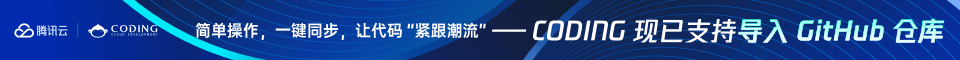Django视频教程，极客时间出口，质量有保证

# PHP For 循环

## for 循环

for 循环用于您预先知道脚本需要运行的次数的情况。

### 语法

for (初始值; 条件; 增量)
{

}

• 初始值：主要是初始化一个变量值，用于设置一个计数器（但可以是任何在循环的开始被执行一次的代码）。
• 条件：循环执行的限制条件。如果为 TRUE，则循环继续。如果为 FALSE，则循环结束。
• 增量：主要用于递增计数器（但可以是任何在循环的结束被执行的代码）。

### 实例

<html>
<body>

<?php
for (\$i=1; \$i<=5; \$i++)
{
echo "The number is " . \$i . "<br>";
}
?>

</body>
</html>

The number is 1
The number is 2
The number is 3
The number is 4
The number is 5

## foreach 循环

foreach 循环用于遍历数组。

### Syntax

foreach (\$array as\$value)
{

}

### 实例

<html>
<body>

<?php
\$x=array("one","two","three");
foreach (\$x as \$value)
{
echo \$value . "<br>";
}
?>

</body>
</html>

one
two
three